# Quant Quiz For IBPS PO Pre Part - 12#### Ques 1.

The average age of 30 students in a class is 16 years. If the age of their teacher is 47 years, what is the average age of the students and the teacher combined ?

(a) 17 2/3 years
(b) 31 1/2 years
(c) 16 years
(d) 17 years
Ans 1. Total age of 30 students = 30 x 16
= 480 years
. . Average age of 30 Students along with the teacher
= 480 + 47 / 30 + 1 = 527/31 = 17 years

#### Ques 2.

Simplify :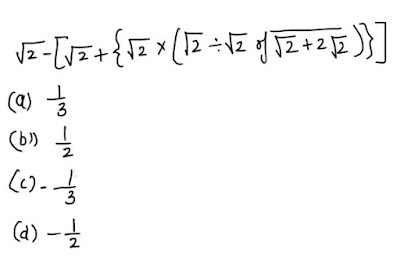Ans 2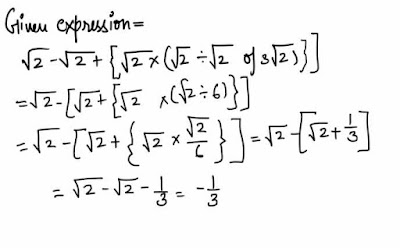#### Ques 3.

The Perimeter of a square , the perimeter of an equilateral triangle and the circumference of a circle are all equal. The maximum area is of
(a) Square
(b) Equilateral
(c) Circle
(d) Nothing can be concluded
Ans 3. Given that 4a = 3x = 2rr,
where a is the side of square , x is the side of equilateral triangle and r is the radius of circle .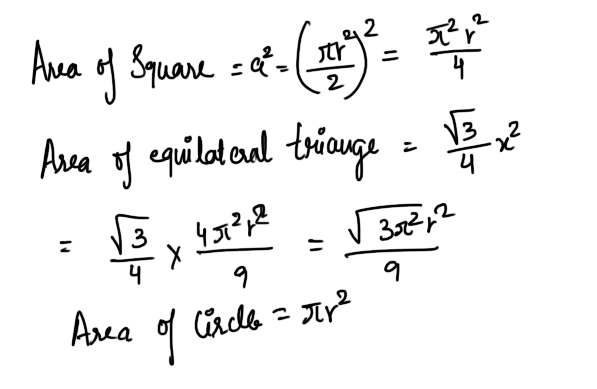#### Ques 4

An electronic device make a beep after every 60 s while another device make a beep after 66 s. If They beeped together at 10 a.m. then the time when they will beep together next time is
(a) 10:11 a.m.
(b) 10 : 13 a.m.
(c) 10: 15 a.m.
(d) 10 : 18 a.m.
Ans 4.  The L.C.M. of 60 and 66 = 660 seconds
= 11 minutes
they beeped together at 10 a.m. hence they would beep together again  after 11 minutes i.e. at 10.11 a.m.

#### Ques 5.

A student was asked to multiply a given number by 8/17 .His answer was 225 more than the correct answer because he divided the number by 8/17 . The given number was
(a) 8
(b) 17
(c) 64
(d) 136
Ans 5.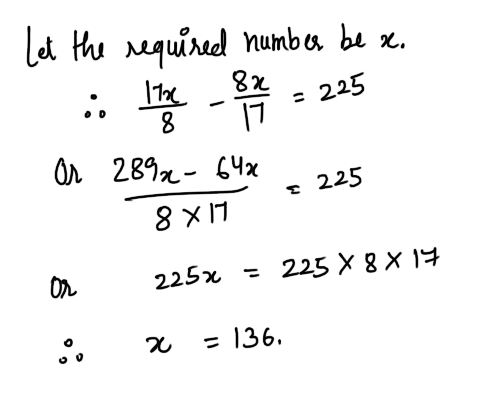#### Ques 6.

If one -eighth of a sick is in mud, one-half of the remaining in water and the remaining 3.5 cm out of water , total length of the stick is
(a) 6 cm
(b) 7 cm
(c) 8 cm
(d) 11 cm
Ans 6.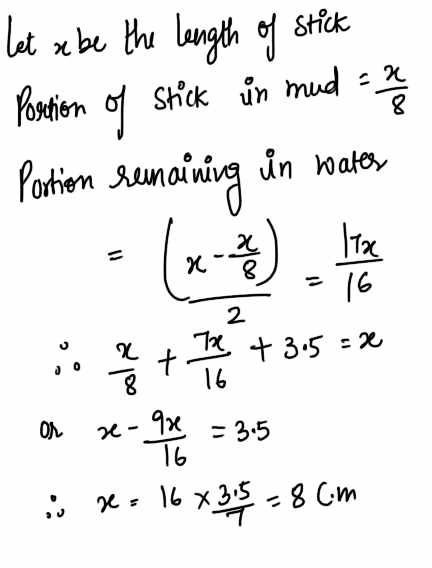#### Ques 7.

The value of /15612+/154+/225 is
(a) 13
(b) 15
(c) 25
(d) 125
Ans 7.#### Ques 8.

/12 + / 12 + /12 + ..... is equal to
(a) 3
(b) 4
(c) 6
(d) greater than 6
Ans 8.#### Ques 9

If 2x=3y= 6-z , then ( 1/x + 1/y + 1/z) is equal to
(a) 0
(b) 1
(c) 3/2
(d) -1/2
Ans 9.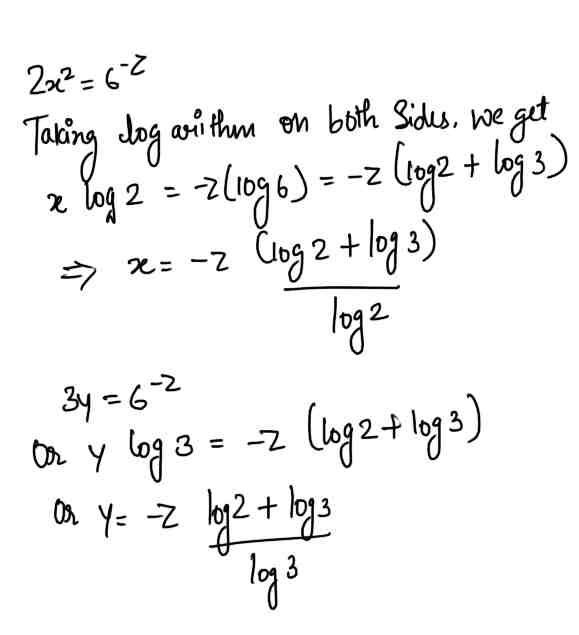#### Ques 10.

If ax = by = cz and b2 = ac , then y is equal to
(a) xz/x +z
(b) xz / 2(x-z)
(c) xz/2(z-x)
(d) 2xz/(x +2)
Ans 10.#### Ques 11.

If the price of sugar decreases by 20% , find by how much per cent must a householder increase her consumption so as not to decrease the expenditure .
(a) 10%
(b) 25%
(c) 18%
(d) 20%
Ans 11.